#### NCERT Solutions for Class 11th: Ch 14 Oscillations Physics Science

Page No: 358

Excercises

14.1. Which of the following examples represent periodic motion?
(a) A swimmer completing one (return) trip from one bank of a river to the other and back.
(b) A freely suspended bar magnet displaced from its N-S direction and released.
(c) A hydrogen molecule rotating about its center of mass.
(d) An arrow released from a bow.

(a) The swimmer's motion is not periodic. Though the motion of a swimmer is to and fro but will not have a definite period.

(b) The motion of a freely-suspended magnet, if displaced from its N-S direction and released, is periodic because the magnet oscillates about its position with a definite period of time.

(c) When a hydrogen molecule rotates about its centre of mass, it comes to the same position again and again after an equal interval of time. Such a motion is periodic.

(d) An arrow released from a bow moves only in the forward direction. It does not come backward. Hence, this motion is not a periodic.

14.2. Which of the following examples represent (nearly) simple harmonic motion and which represent periodic but not simple harmonic motion?
(a) the rotation of earth about its axis.
(b) motion of an oscillating mercury column in a U-tube.
(c) motion of a ball bearing inside a smooth curved bowl, when released from a point slightly above the lower most point.
(d) general vibrations of a polyatomic molecule about its equilibrium position.

(a) It is priodic but not simple harmonic motion because it is not to and fro about a fixed point.

(b) It is a simple harmonic motion because the mercury moves to and fro on the same path, about the fixed position, with a certain period of time.

(c) It is simple harmonic motion because the ball moves to and fro about the lowermost point of the bowl when released. Also, the ball comes back to its initial position in the same period of time, again and again.

(d) A polyatomic molecule has many natural frequencies of oscillation. Its vibration is the superposition of individual simple harmonic motions of a number of different molecules. Hence, it is not simple harmonic, but periodic.

14.3. Figure 14.27 depicts four x-t plots for linear motion of a particle. Which of the plots represent periodic motion? What is the period of motion (in case of periodic motion)?

(a) It is not a periodic motion. This represents a unidirectional, linear uniform motion. There is no repetition of motion in this case.

(b) In this case, the motion of the particle repeats itself after 2 s. Hence, it is a periodic motion, having a period of 2 s.

(c) It is not a periodic motion. This is because the particle repeats the motion in one position only. For a periodic motion, the entire motion of the particle must be repeated in equal intervals of time.

(d) In this case, the motion of the particle repeats itself after 2 s. Hence, it is a periodic motion, having a period of 2 s.

Page No: 359

14.4. Which of the following functions of time represent (a) simple harmonic, (b) periodic but not simple harmonic, and (c) non-periodic motion? Give period for each case of periodic motion (ω is any positive constant):
(a) sin ωt – cos ωt
(b) sin3 ωt
(c) 3 cos (π/4 – 2ωt)
(d) cos ωt + cos 3ωt + cos 5ωt
(e) exp (–ω2t2)

(a) SHM
The given function is:
sin ω– cos ωt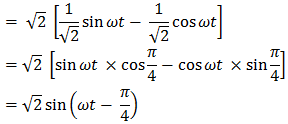This function represents SHM as it can be written in the form: a sin (ωt + Φ)
Its period is: 2π/ω

(b) Periodic but not SHM
The given function is:
sin3 ωt = 1/4 [3 sin ωt - sin3ωt]
The terms sin ωt and sin ωt individually represent simple harmonic motion (SHM). However, the superposition of two SHM is periodic and not simple harmonic.
Ites period is: 2π/ω

(c) SHM
The given function is: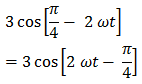This function represents simple harmonic motion because it can be written in the form: a cos (ωt + Φ)Its period is: 2π/2ω = π/ω

(d) Periodic, but not SHM
The given function  is cosωt + cos3ωt + cos5ωt. Each individual cosine function represents SHM. However, the superposition of three simple harmonic motions is periodic, but not simple harmonic.

(e) Non-periodic motion
The given function exp(-ω2t2) is an exponential function. Exponential functions do not repeat themselves. Therefore, it is a non-periodic motion.

(f) The given function 1 + ωt + ω2t2 is non-periodic.

14.5. A particle is in linear simple harmonic motion between two points, A and B, 10 cm apart. Take the direction from A to B as the positive direction and give the signs of velocity, acceleration and force on the particle when it is
(a) at the end A,
(b) at the end B,
(c) at the mid-point of AB going towards A,
(d) at 2 cm away from B going towards A,
(e) at 3 cm away from A going towards B, and
(f) at 4 cm away from B going towards A.From above figure, where A and B represent the two extreme positions of a SHM. FOr velocity, the direction from A to B is taken to b positive. The acceleration and the force, along AP are taken as positive and alsong Bp are taken as negative.

(a) At the end A, the particle executing SHM is momentarily at rest being its extreme position of motion. Therefore, its velocity is zero. Acceleration is positive becaue it is directed along AP, Force is also Positive since the force is directed along AP.

(b) At the end B, velocity is zero. Here, accleration and force are negative as they are directed along BP.

(c) At the mid point of AB going towards A, the particle is at its mean psoiton P, with a tendancy to move along PA. Hence, velocity is positive. Both accleration and force are zero.

(d) At 2 cm away from B going towards A, the particle is at Q, with a tendancy to move along QP, which is negative direction. Therefore,  velocity, acceleration and force all are positive.

(e) At 3 sm away from A going towards B, the particle is at R, with a tendancy to move along RP, which is positive direction. Here, velocity, acceleration all are positive.

(f) At 4 cm away from A going towards A, the particles is at S, with a tendancy to move along SA, which is negative direction. Therefore, velocity is negative but acclereation is directed towards mean position, along SP. Hence it is positive and also force is positive similarly.

14.6. Which of the following relationships between the acceleration a and the displacement x of a particle involve simple harmonic motion?
(a) a = 0.7x
(b) a = –200x2
(c) a = –10x
(d) a = 100x3

In SHM, acceleration a is related to displacement by the relation of the form a = -kx, which is for relation (c).

14.7. The motion of a particle executing simple harmonic motion is described by the displacement function,
x (t) = A cos (ωt + φ).
If the initial (t = 0) position of the particle is 1 cm and its initial velocity is ω cm/s, what are its amplitude and initial phase angle? The angular frequency of the particle is π s–1. If instead of the cosine function, we choose the sine function to describe the SHM: x = B sin (ωt + α), what are the amplitude and initial phase of the particle with the above initial conditions.

Intially, at t = 0;
Displacement, x = 1 cm
Intial velocity, v = ω cm/ sec.
Angular frequency, ω = π rad/s–1
It is given that,
x(t) = A cos(ωt + Φ)
1 = A cos(ω × 0 + Φ) = A cos Φ
A cosΦ = 1                          ...(i)
Velocity, v= dx/dt
ω = -A ωsin(ωt + Φ)
1 = -A sin(ω × 0 + Φ) = -A sin Φ
sin Φ = -1                         ...(ii)

Squaring and adding equations (i) and (ii), we get:
A2 (sin2 Φ + cos2 Φ) = 1 + 1
A2 = 2
A = √2 cm
Dividing equation (ii) by equation (i), we get:
tanΦ = -1
∴ Φ = 3π/4 , 7π/4,......

SHM is given as:
x = Bsin (ωt + α)
Putting the given values in this equation, we get:
1 = Bsin[ω × 0 + α] = 1 + 1
Bsin α = 1                           ...(iii)
Velocity, v = ωBcos (ωt + α)
Substituting the given values, we get:
π = πBsin α
Bsin α = 1                            ...(iv)

Squaring and adding equations (iii) and (iv), we get:
B2 [sin2 α + cos2 α] = 1 + 1
B2 = 2
B = √2 cm
Dividing equation (iii) by equation (iv), we get:
Bsin α / Bcos α = 1/1
tan α = 1 = tan π/4

∴ α = π/4, 5π/4,......

14.8. A spring balance has a scale that reads from 0 to 50 kg. The length of the scale is 20 cm. A body suspended from this balance, when displaced and released, oscillates with a period of 0.6 s. What is the weight of the body?

Maximum mass that the scale can read, M = 50 kg
Maximum displacement of the spring = Length of the scale, l = 20 cm = 0.2 m
Time period, T = 0.6 s
Maximum force exerted on the spring, F = Mg
where,
g = acceleration due to gravity = 9.8 m/s2
F = 50 × 9.8 = 490
∴  Spring constant, k = F/l = 490/0.2 = 2450 N m-1.
Mass m, is suspended from the balance.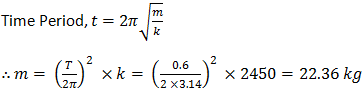∴Weight of the body = mg = 22.36 × 9.8 = 219.167 N
Hence, the weight of the body is about 219 N.

14.9. A spring having with a spring constant 1200 N m–1 is mounted on a horizontal table as shown in Fig. A mass of 3 kg is attached to the free end of the spring. The mass is then pulled sideways to a distance of 2.0 cm and released.Determine (i) the frequency of oscillations, (ii) maximum acceleration of the mass, and (iii) the maximum speed of the mass.

Spring constant, k = 1200 N m–1
Mass, m = 3 kg
Displacement, A = 2.0 cm = 0.02 cm

(i) Frequency of oscillation v, is given by the relation:Hence, the frequency of oscillations is 3.18 cycles per second.

(ii) Maximum acceleration (a) is given by the relation:
a = ω2 A
where,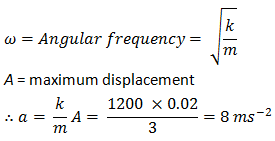Hence, the maximum acceleration of the mass is 8.0 m/s2.

(iii) Maximum velocity, vmax = AωHence, the maximum velocity of the mass is 0.4 m/s.

14.10. In Exercise 14.9, let us take the position of mass when the spring is unstreched as x = 0, and the direction from left to right as the positive direction of x-axis. Give x as a function of time t for the oscillating mass if at the moment we start the stopwatch (t = 0), the mass is
(a) at the mean position,
(b) at the maximum stretched position, and
(c) at the maximum compressed position.
In what way do these functions for SHM differ from each other, in frequency, in amplitude or the initial phase?

Distance travelled by the mass sideways, a = 2.0 cm
Angular frequency of oscillation: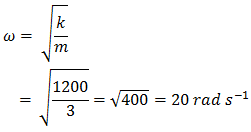(a) As time is noted from the mean position, hence using
x = a sin ω t, we have x = 2 sin 20 t

(b) At maximum stretched position, the body is at the extreme right position, with an intial phase of π/2 rad. Then,(c) At maximum compressed position, the body is at left position, with an intial phase of 3 π/2 rad. Then,The functions neither differ in amplitude nor in frequency. They differ in intial phase.

Page No: 360

14.11. Figures 14.29 correspond to two circular motions. The radius of the circle, the period of revolution, the initial position, and the sense of revolution (i.e. clockwise or anti-clockwise) are indicated on each figure.Obtain the corresponding simple harmonic motions of the x-projection of the radius vector of the revolving particle P, in each case.

(a) Time period, t = 2 s
Amplitude, A = 3 cm
At time, t = 0, the radius vector OP makes an angle π/2 with the positive x-axis,.e., phase angle Φ = +π/2
Therefore, the equation of simple harmonic motion for the x-projection of OP, at the time t, is given by the displacement equation: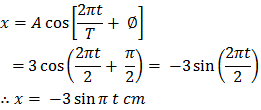(b) Time Period, t = 4 s
Amplitude, a = 2 m
At time t = 0, OP makes an angle π with the x-axis, in the anticlockwise direction, Hence, phase angle Φ = +π
Therefore, the equation of simple harmonic motion for the x-projection of OP, at the time t, is given as:14.12. Plot the corresponding reference circle for each of the following simple harmonic motions. Indicate the initial (t = 0) position of the particle, the radius of the circle, and the angular speed of the rotating particle. For simplicity, the sense of rotation may be fixed to be anticlockwise in every case: (x is in cm and t is in s).
(a) x = –2 sin (3t + π/3)
(b) x = cos (π/6 – t)
(c) x = 3 sin (2πt + π/4)
(d) x = 2 cos πt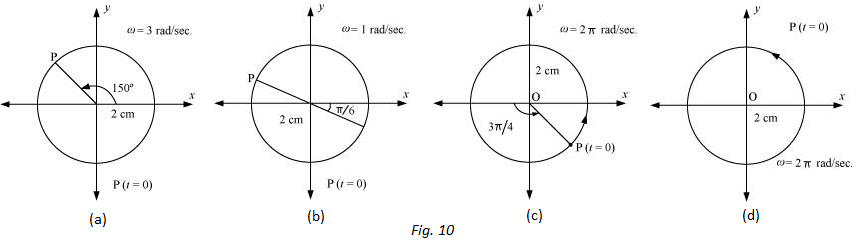(a)Amplitude, A = 2 cm
Phase angle, Φ = 5π/6 = 150°.
Angular velocity = ω = 2π/T = 3rad/sec.
The motion of the particle can be plotted as shown in fig. 10(a).

(b)Amplitude, A = 1
Phase angle, Φ = -π/6 = -30°.
Angular velocity, ω = 2π/T = 1 rad/s.
The motion of the particle can be plotted as shown in fig. 10(b).

(c)Amplitude, A = 3 cm
Phase angle, Φ = 3π/4 = 135°
Angular velocity, ω = 2π/T = 2 rad/s.
The motion of the particle can be plotted as shown in fig. 10(c).

(d)Amplitude, A = 2 cm
Phase angle, Φ = 0
Angular velocity, ω = π rad/s.
The motion of the particle can be plotted as shown in fig. 10(d).

14.13. Figure 14.30 (a) shows a spring of force constant k clamped rigidly at one end and a mass m attached to its free end. A force F applied at the free end stretches the spring. Figure 14.30 (b) shows the same spring with both ends free and attached to a mass m at either end. Each end of the spring in Fig. 14.30(b) is stretched by the same force F.(a) What is the maximum extension of the spring in the two cases?
(b) If the mass in Fig. (a) and the two masses in Fig. (b) are released, what is the period of oscillation in each case?

(a) The maximum extension of the spring in both cases will = Flk, where k is the spring constant of the springs used.

(b) In Fig.14.30(a) if x is the extension in the spring, when mass m is returning to its mean position after being released free, then restoring force on the mass iss F = -kx, i.e., F x
As, this F is directed towards mean position of the mass, hence the mass attached to the spring will execute SHM.
Spring factor = spring constant = k
inertia factor = mass of the given mass = m
As time period,In Fig.14.30(b), we have a two body system of spring constant k and reduced mass, µ = m × m / m + m = m/2.
Inertia factor = m/2
Spring factor = k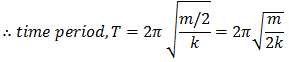Page No: 361

14.14. The piston in the cylinder head of a locomotive has a stroke (twice the amplitude) of 1.0 m. If the piston moves with simple harmonic motion with an angular frequency of 200 rad/min, what is its maximum speed?

Angular frequency of the piston, ω = 200 rad/ min.
Stroke = 1.0 m
Amplitude, A = 1.0/2 = 0.5 m
The maximum speed (vmax) of piston is given by the relation:
vmax = Aω = 200 × 0.5 = 100m/min.

14.15. The acceleration due to gravity on the surface of moon is 1.7 ms–2. What is the time period of a simple pendulum on the surface of moon if its time period on the surface of earth is 3.5 s? (g on the surface of earth is 9.8 ms–2)

Acceleration due to gravity on the surface of moon,g' = 1.7 m s–2
Acceleration due to gravity on the surface of earth, g = 9.8 m s–2
Time period of a simple pendulum on earth, T = 3.5 sHence, the time period of the simple pendulum on the surface of moon is 8.4 s.

(a) Time period of a particle in SHM depends on the force constant k and mass m of the particle:
T = 2π √m/k. A simple pendulum executes SHM approximately. Why then is the time period of a pendulum independent of the mass of the pendulum?
(b) The motion of a simple pendulum is approximately simple harmonic for small angle oscillations. For larger angles of oscillation, a more involved analysis shows that T is greater than 2π √l/g
Think of a qualitative argument to appreciate this result.
(c) A man with a wristwatch on his hand falls from the top of a tower. Does the watch give correct time during the free fall?
(d) What is the frequency of oscillation of a simple pendulum mounted in a cabinthat is freely falling under gravity?

(a) For a simple pendulum, force constant or spring factor k is proportional to mass m, therefore, m cancels out in denominator as well as in numerator. That is why the time period of simple pendulum is independent of the mass of the bob.

(b) In the case of a simple pendulum, the restoring force acting on the bob of the pendulum is given as:
F = –mg sinθ
where,
F = Restoring force
m = Mass of the bob
g = Acceleration due to gravity
θ = Angle of displacement
For small θ, sinθ ≈ θ
For large θ, sinθ is greater than θ.
This decreases the effective value of g.
Hence, the time period increases as:
T = 2π √l/g

(c) Yes, because the working of the wrist watch depends on spring action and it has nothing to do with gravity.
(d) Gravity disappears for a man under free fall, so frequency is zero.

14.17. A simple pendulum of length l and having a bob of mass M is suspended in a car. The car is moving on a circular track of radius R with a uniform speed v. If the pendulum makes small oscillations in a radial direction about its equilibrium position, what will be its time period?

The bob of the simple pendulum will experience the acceleration due to gravity and the centripetal acceleration provided by the circular motion of the car.
Acceleration due to gravity = g
Centripetal acceleration = v2/R
where,
v is the uniform speed of the car
R is the radius of the track
Effective acceleration (g') is given as: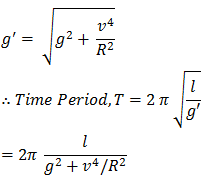14.18. Cylindrical piece of cork of density of base area A and height h floats in a liquid of density ρ1. The cork is depressed slightly and then released. Show that the cork oscillates up and down simple harmonically with a periodwhere ρ is the density of cork. (Ignore damping due to viscosity of the liquid).

Base area of the cork = A
Height of the cork = h
Density of the liquid = ρ1
Density of the cork = ρ

In equilibrium:
Weight of the cork = Weight of the liquid displaced by the floating cork
Let the cork be depressed slightly by x. As a result, some extra water of a certain volume is displaced. Hence, an extra up-thrust acts upward and provides the restoring force to the cork.
Up-thrust = Restoring force, F = Weight of the extra water displaced
F = ­–(Volume × Density × g)
Volume = Area × Distance through which the cork is depressed
Volume = Ax
F = – A ρg .....(i)
Accroding to the force law:
F = kx
k = F/x
where, k is constant
k = F/x = -Aρg ....(ii)
The time period of the oscillations of the cork:
T = 2π √m/     ....(iii)
where,
m = Mass of the cork
= Volume of the cork × Density
= Base area of the cork × Height of the cork × Density of the cork
= Ahρ
Hence, the expression for the time period becomes: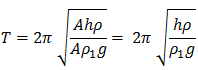14.19. One end of a U-tube containing mercury is connected to a suction pump and the other end to atmosphere. A small pressure difference is maintained between the two columns. Show that, when the suction pump is removed, the column of mercury in the U-tube executes simple harmonic motion.

Area of cross-section of the U-tube = A
Density of the mercury column = ρ
Acceleration due to gravity = g
Restoring force, F = Weight of the mercury column of a certain height
F = –(Volume × Density × g)
F = –(A × 2h × ρ × g) = –2Aρgh = –k × Displacement in one of the arms (h)
Where,
2h is the height of the mercury column in the two arms
k is a constant, given by k =  -F/h = 2Aρgwhere,
m is the mass of the mercury column
Let l be the length of the total mercury in the U-tube.
Mass of mercury, m = Volume of mercury × Density of mercury = AlρHence, the mercury column executes simple harmonic motion with time period 2π √l/2g .

14.20. An air chamber of volume V has a neck area of cross section a into which a ball of mass m just fits and can move up and down without any friction (Fig.14.33). Show that when the ball is pressed down a little and released, it executes SHM. Obtain an expression for the time period of oscillations assuming pressure-volume variations of air to be isothermal [see Fig. 14.33].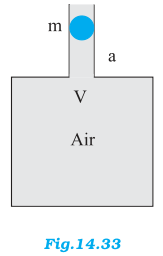Volume of the air chamber = V
Area of cross-section of the neck = a
Mass of the ball = m
The pressure inside the chamber is equal to the atmospheric pressure.
Let the ball be depressed by x units. As a result of this depression, there would be a decrease in the volume and an increase in the pressure inside the chamber.
Decrease in the volume of the air chamber, ΔV = ax
Volumetric strain = Change in volume/original volume
⇒ ΔV/V = ax/V
Bulk modulus of air, B = Stress/Strain = -p/ax/V
In this case, stress is the increase in pressure. The negative sign indicates that pressure increases with decrease in volume.
p = -Bax/V
The restoring force acting on the ball, F = p × a
= -Bax/V .a
= -Bax2/V ......(i)
In simple harmonic motion, the equation for restoring force is:
F = -kx       .....(ii)
where, k is the spring constant
Comparing equations (i) and (ii), we get:
k = Ba2/V
Time Period,Page No: 362

14.21. You are riding in an automobile of mass 3000 kg. Assuming that you are examining the oscillation characteristics of its suspension system. The suspension sags 15 cm when the entire automobile is placed on it. Also, the amplitude of oscillation decreases by 50% during one complete oscillation. Estimate the values of (a) the spring constant k and (b) the damping constant b for the spring and shock absorber system of one wheel, assuming that each wheel supports 750 kg.

(a) Mass of the automobile, m = 3000 kg
Displacement in the suspension system, x = 15 cm = 0.15 m
There are 4 springs in parallel to the support of the mass of the automobile.
The equation for the restoring force for the system:
F = –4kx = mg
Where, k is the spring constant of the suspension system
Time period, T = 2π √m/4k
and k = mg/4x = 3000 × 10/ 4 × 0.15 = 5000 = 5 × 104 Nm
Spring Constant, k = 5 × 104 Nm

(b) Each wheel supports a mass, M = 3000/4 = 750 kg
For damping factor b, the equation for displacement is written as:
x = x0e-bt/2M

The amplitude of oscilliation decreases by 50 %.
x = x0/2
x0/2 = x0e-bt/2M

loge2 = bt/2M
b = 2loge2 / t
where,14.22. Show that for a particle in linear SHM the average kinetic energy over a period of oscillation equals the average potential energy over the same period.

The equation of displacement of a particle executing SHM at an instant t is given as:
x = Asin ωt
where,
A = Amplitude of oscillation
ω = Angular frequency = √k/M
The velocity of the particle is:v = dx/dt = Aωcosωt
The kinetic energy of the particle is:
Ek = 1/2 Mv2 = 1/2 MA2 ω2 cos2 ωt
The portential energy of the particle is:
Ep = 1/2 kx2 = 1/2 M2 ω2 A2 sin2 ωt

For time period T, the average kinetic energy over a single cycle is given as:And, average potential energy over one cycle is given as:It can be inferred from equations (i) and (ii) that the average kinetic energy for a given time period is equal to the average potential energy for the same time period.

14.23. A circular disc of mass 10 kg is suspended by a wire attached to its centre. The wire is twisted by rotating the disc and released. The period of torsional oscillations is found to be 1.5 s. The radius of the disc is 15 cm. Determine the torsional spring constant of the wire. (Torsional spring constant α is defined by the relation J = –α θ, where J is the restoring couple and θ the angle of twist).

Mass of the circular disc, m = 10 kg
Radius of the disc, r = 15 cm = 0.15 m
The torsional oscillations of the disc has a time period, T = 1.5 s
The moment of inertia of the disc is:
I = 1/2mr2
= 1/2 × (10) × (0.15)2
= 0.1125 kg/m2
Time Period, T = 2π √I
α is the torisonal constant.
α = 4π2 I / T2
= 4 × (π)2 × 0.1125 / (1.5)2
Hence, the torsional spring constant of the wire is 1.972 Nm rad–1.

14.24. A body describes simple harmonic motion with amplitude of 5 cm and a period of 0.2 s. Find the acceleration and velocity of the body when the displacement is (a) 5 cm, (b) 3 cm, (c) 0 cm.

r = 5 cm = 0.05 m
T = 0.2 s
ω = 2π/T = 2π/0.2 = 10 π rad/s

When displacement is y, then acceleration, A = -ω2 y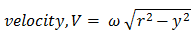Case (a) When y = 5cm = 0.05 m
A = -(10π)2 × 0.05 = -5π2 m/s2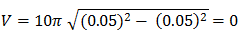Case (b) When y = 3 cm = 0.03 m
A = -(10π)2 × 0.03 = -3π2 m/s2Case (c) When y = 0
A = -(10π)2 × 0 = 014.25. A mass attached to a spring is free to oscillate, with angular velocity ω, in a horizontal plane without friction or damping. It is pulled to a distance x0 and pushed towards the centre with a velocity v0 at time t = 0. Determine the amplitude of the resulting oscillations in terms of the parameters ω, x0 and v0. [Hint: Start with the equation x = a cos (ωt) and note that the initial velocity is negative.]

The displacement rquation for an oscillating mass is given by:
x = Acos(ωt + θ)
where,
A is the amplitude
x is the displacement
θ is the phase constant

Velcoity, v = dx/dt = -Aωsin(ωt + θ)
At t = 0, x = x0
x0 = Acos θ = x0    ....(i)
and, dx/dt = -v0Aωsinθ
Asinθ = v0/ω          ...(ii)

Squaring and adding equations (i) and (ii), we get: